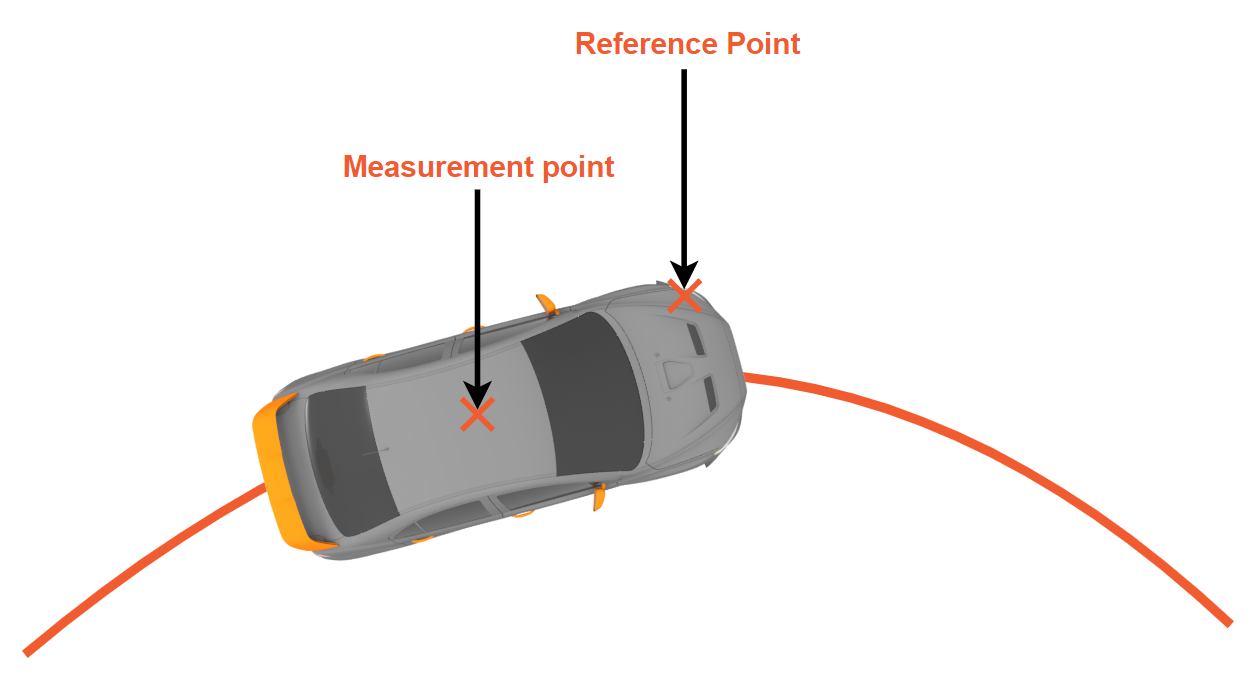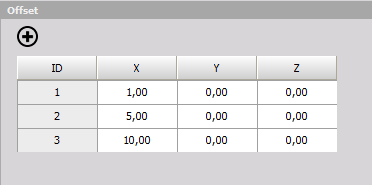# Reference Point

The reference point processing plugin calculates position, velocity and acceleration of a point that is offset from an initial point of measurement.### Inputs

• Velocity (X, Y, Z)
• Angular Velocity (X, Y, Z)
• Acceleration (X, Y, Z)

The navigation channel consists of geodetic position (latitude, longitude, altitude) and orientation (roll, pitch, yaw).

### Outputs